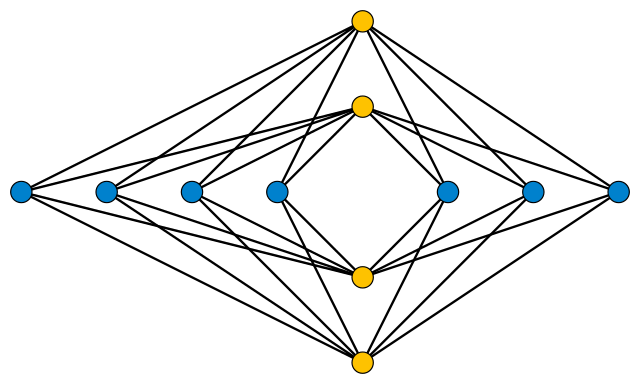# Turán’s Brick Factory ProblemDuring World War II, Hungarian mathematician Pál Turán was forced to work in a brick factory. His job was to push a wagonload of bricks along a track from a kiln to storage site. The factory contained several kilns and storage sites, with tracks criss-crossing the floor among them. Turán found it difficult to push the wagon across a track crossing, and in his mind he began to consider how the factory might be redesigned to minimize these crossings.

After the war, Turán mentioned the problem in talks in Poland, and mathematicians Kazimierz Zarankiewicz and Kazimierz Urbanik both took it up. They showed that it’s always possible to complete the layout as shown above, with the kilns along one axis and the storage sites along the other, each group arranged as evenly as possible around the origin, with the tracks running as straight lines between each possible pair. The number of crossings, then, is$\displaystyle \mathrm{cr}\left ( K_{m,n} \right ) \leq \left \lfloor \frac{n}{2} \right \rfloor \left \lfloor \frac{n-1}{2} \right \rfloor \left \lfloor \frac{m}{2} \right \rfloor \left \lfloor \frac{m-1}{2} \right \rfloor ,$

where m and n are the number of kilns and storage sites and$\displaystyle \left \lfloor \right \rfloor$ denotes the floor function, which just means that we take the greatest integer less than the value in brackets. In the case of 4 kilns and 7 storage sites, that gives us$\displaystyle \left \lfloor \frac{7}{2} \right \rfloor \left \lfloor \frac{7-1}{2} \right \rfloor \left \lfloor \frac{4}{2} \right \rfloor \left \lfloor \frac{4-1}{2} \right \rfloor = 18 ,$

which is the number of crossings in the diagram above.

Is that the best we can do? No one knows. Zarankiewicz and Urbanik thought that their formula gave the fewest possible crossings, but their proof was found to be erroneous 11 years later. Whether a factory can be designed whose layout contains fewer crossings remains an open problem.